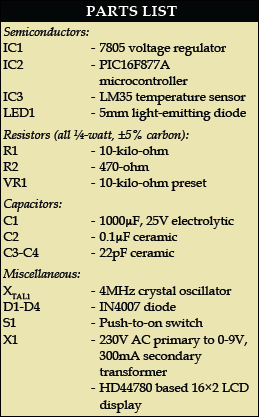Temperature monitoring and control is important in industry environments. Sensors are widely used for measurement of temperature. Usually, a temperature sensor converts the temperature into an equivalent voltage output. IC LM35 is such a sensor. Here we describe a simple temperature measurement and display system based on LM35 sensor and PIC16F877A microcontroller. The temperature in degrees Celsius is displayed on a 16×2 LCD.

Fig.1 shows the functional block diagram of the PIC16F877A-based temperature monitoring system. The key features of this system are:

1. Continuous monitoring of temperature with 1-second update interval (which can be varied in the program).

2. Temperature measurement using LM35 precision integrated-circuit sensor.

3. Precise analogue-to-digital conversion using in-built 10-bit analogue- to-digital converter (ADC) of PIC16F877A microcontroller.

Hardware description

Fig.2.shows the circuit of the temperature monitoring system. The circuit mainly consists of the LM35 temperature sensor, PIC16F877A microcontroller and HD44780 controller based 16×2 LCD.The output of the sensor is fed to the internal ADC of the microcontroller. Pin 2 of the microcontroller (RA0/AN0) is channel-1 of the internal ADC. The analogue voltage output of the sensor is converted into its equivalent digital value by the ADC and then its equivalent degree Celsius value is calculated by the software. The calculated temperature value is displayed on the LCD.

LM35 sensor. Fig.3 shows the pin configuration of LM35. It is a precision integrated-circuit centigrade temperature sensor whose output voltage is linearly proportional to the Celsius (Centigrade) temperature. The LM35 thus has an advantage over linear temperature sensors calibrated in degree Kelvin, as the user is not required to subtract a large constant voltage from its output to obtain convenient Centigrade scaling. For each degree Celsius change in temperature, the sensor output changes by 10 mV.

The sensor can measure temperature in the range of 0 to 100°C, i.e., the output of the sensor varies from 0 to 1000 mV. The LM35 operates over the temperature range of -55° to +150°C, while the LM35C is rated for a -40°C to +110°C range (-10°C with improved accuracy).

Pin layout of the sensor is as follows:

Pin 1-VDD
Pin 2-Output of the sensor
Pin 3-VSS

PIC16F877A microcontroller. IC PIC16F877A is an 8-bit microcontroller with 8k×14-bit flash program memory, 368 bytes of RAM and many other extra peripherals like ADC, universal synchronous asynchronous receiver transmitter, master synchronous serial port, timers, compare capture and pulse-width modulation modules, and analogue comparators. It is based on the reduced instruction set computer (RISC) architecture.

The microcontroller processes the sensor output to compute the temperature in degree Celsius. The internal ADC of the microcontroller is used to convert the analogue output of the sensor into its equivalent digital value.

The internal ADC of the microcontroller has eight channels of analogue input and gives 10-bit digital output. In this project, the reference voltage to the ADC is the same as the supply voltage to the microcontroller, i.e., 5V. The resolution of the ADC can be calculated as follows:

Resolution = Vref / (1024-1) …(as it is a 10-bit ADC)
= 5/1023
= 4.887 mV

It means that for 4.887mV change in the analogue input, the ADC output changes by binary ‘1’ with a reference voltage of 5V.

Analogue output of the sensor at its pin 2 is connected to Port A at RA0 for conversion into digital equivalent.

The LCD. A 16×2 LCD based on HD44780 controller is used for displaying the temperature. The control lines EN, R/W and RS of the LCD module are connected to pins RA1, RA2 and RA3 of Port A of the microcontroller, respectively. The commands and the data to be displayed are sent to the LCD module in the nibble mode from Port D of the microcontroller. The higher four bits of the LCD (D4 through D7) are connected to the lower nibble of Port D (RD0 through RD3).

Software description

The software code is written in ‘C’ language and compiled using Hitech C cross compiler in MPLAB IDE. The software performs the following operations in an infinite loop:

1. Initiate analogue-to-digital conversion and obtain the result

2. Calculate the equivalent voltage value from the ADC result

3. Calculate the temperature in degree Celsius from the voltage value

4. Display the temperature on the LCD

•Abhimanyu Rathore

We have updated the source code. Please check.

1.rmin

do u implement it physically?

2.efylab

Yes, the code was tested on hardware

3.Rumen

4.karthik

super done job good job…but i have small doubt of that code ..what is the need of ch4 and f1=num-temp.everytime f1 will be zero.

5.Methembe

where do i find the code?

•EFY Team

The source code is present on the last page of the article.

6.Praveen

Where did I get protecus modle of this sensor